# 978-0134292380 Chapter 2 Part 1

Document Type
Test Prep
Book Title
Fundamentals of Hydraulic Engineering Systems 5th Edition
Authors
A. Osman H. Akan, Ned H. C. Hwang, Robert J. Houghtalen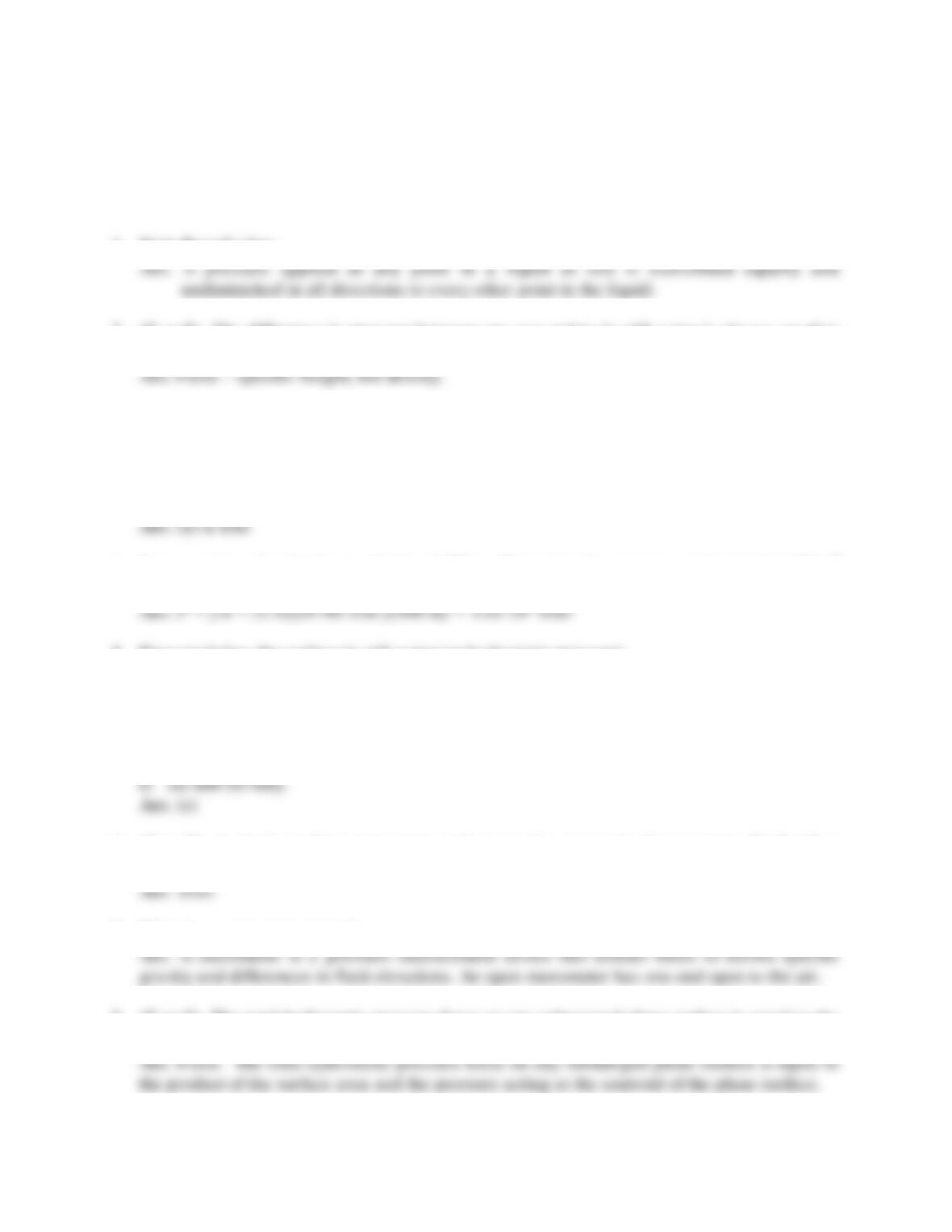6
TEST QUESTIONS - CHAPTER #2
1. State Pascal’s law.
2. (T or F) The difference in pressure between any two points in still water is always equal to
the product of the density of water and the difference in elevation between the two points.
3. Gage pressure is defined as
a) the pressure measured above atmospheric pressure.
b) the pressure measured plus atmospheric pressure.
c) the difference in pressure between two points.
d) pressure expressed in terms of the height of a water column.
4. Some species of seals dive to depths of 400 m. Determine the pressure at that depth in N/m2
assuming sea water has a specific gravity of 1.03.
5. Pressure below the surface in still water (or hydrostatic pressure)
a) is linearly related to depth.
b) acts normal (perpendicular) to any solid surface.
c) is related to the temperature of the fluid.
d) at a given depth, will act equally in any direction.
e) all of the above.
6. (T or F) A single-reading manometer makes use of a reservoir of manometry fluid with a
large cross sectional area so that pressure calculations are only based on one reading.
7. What is an open manometer?
8. (T or F) The total hydrostatic pressure force on any submerged plane surface is equal to the
product of the surface area and the pressure acting at the center of pressure of the surface.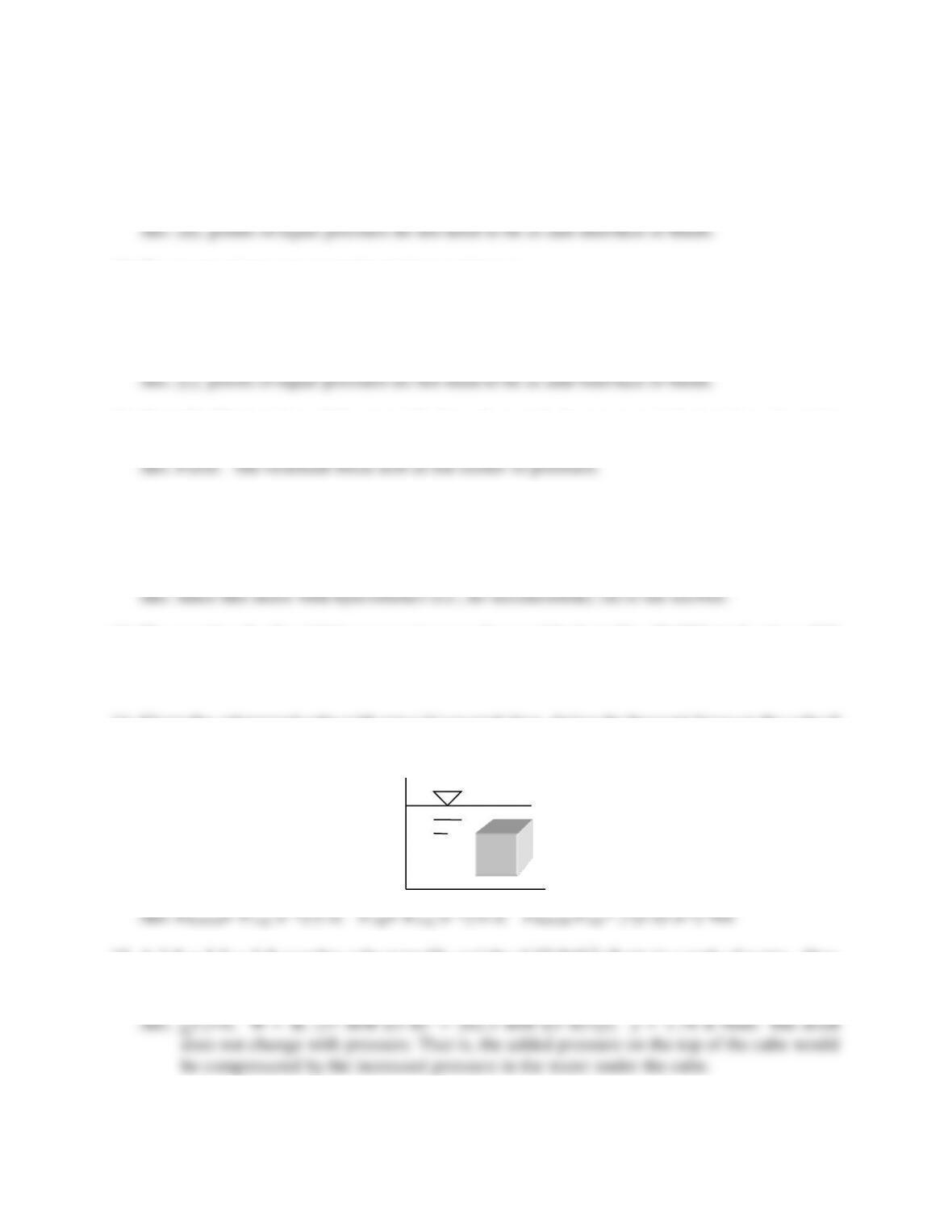7
9. A surface of equal pressure requires all of the following except:
a) points of equal pressure must be at the same elevation.
b) points of equal pressure must be in the same fluid.
c) points of equal pressure must be interconnected.
d) points of equal pressure must be at the interface of immiscible fluids.
10. The center of pressure on inclined plane surfaces is:
a) at the centroid.
b) is always above the centroid.
c) is always below the centroid.
d) is not related to the centroid.
11. (T or F) The location of the centroid of a submerged plane area and the location where the
resultant pressure force acts on that area are identical.
12. The equation for the determination of a hydrostatic force on a plane surface and its location
are derived using all of the following concepts except
a) integration of the pressure equation b) moment of inertia concept
c) principle of moments d) Newton’s 2nd Law
13. The equation for the righting moment on a submerged body is M = W·GM·sin θ, where GM
= MB GB or MB + GB. Under what conditions is the sum used instead of the difference?
Ans. Use the sum when the center of gravity is below the center of buoyancy.
14. Given the submerged cube with area (A) on each face, derive the buoyant force on the cube if
the depth (below the surface of the water) to the top of the cube is x and the depth to the
bottom of the cube is y. Show all steps.
15. A 3 ft x 3 ft x 3 ft wooden cube (specific weight of 37 lb/ft3) floats in a tank of water. How
much of the cube extends above the water surface? If the tank were pressurized to 2 atm
(29.4 psi), how much of the cube would extend above the water surface? Explain.8
16. The derivation of the flotation stability equation utilizes which principles? Note: More than
a) moment of a force couple b) moment of inertia
c) Newton’s 2nd Law d) buoyancy
17. Rotational stability is a major concern in naval engineering. Draw the cross section of the
hull of a ship and label the three important points (i.e., centers) which affect rotational
18. A 4 m (length) by 3 m (width) by 2 m (height) homogeneous box floats with a draft 1.4 m.
What is the distance between the center of buoyancy and the center of gravity?
Ans. G is 1 m up from bottom and B is 0.7 m up from bottom. Thus, GB = 0.3 m.
19. Determine the waterline moment of inertia about the width of a barge (i.e., used to assess
20. (T or F) Floatation stability is dependent on the relative positions of the center of gravity and
the center of buoyancy.
Ans. True.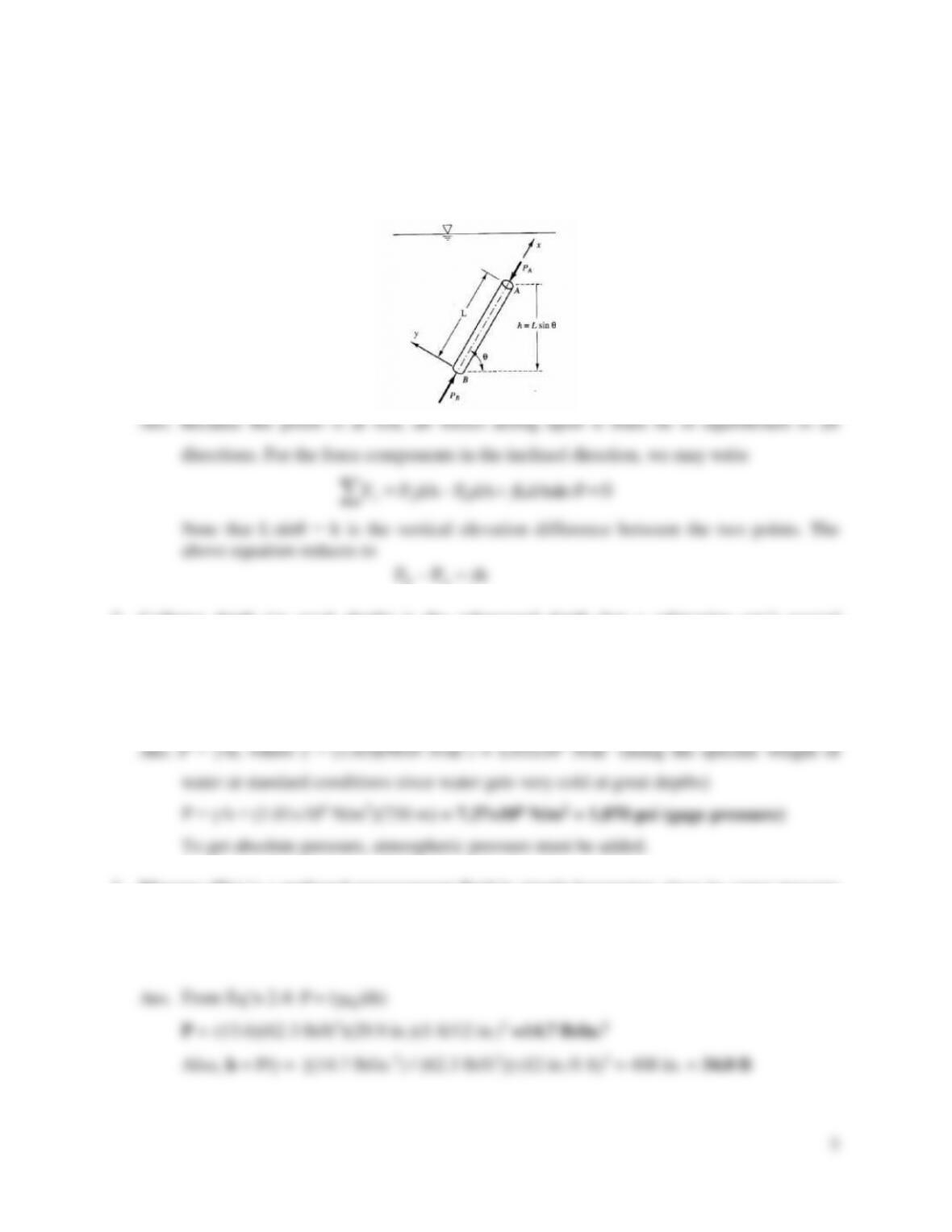9
Problems
1. Given the submerged, inclined rod with a top and bottom area (dA), a length of L, and an
angle of incline of θ, derive an expression that relates the pressure on the top of the rod to the
pressure on the bottom. Show all steps and define all variables.
2. Collapse depth (or crush depth) is the submerged depth that a submarine can’t exceed
without collapsing due to the surrounding water pressure. The collapse depth of modern
submarines is not quite a kilometer (730 m). Assuming sea water to be incompressible (S.G.
= 1.03), what is the crush depth pressure in N/m2 and psi (lb/in2). Is the pressure you
computed absolute or gage pressure?
3. Mercury (Hg) is a preferred measurement fluid in simple barometers since its vapor pressure
is low enough to be ignored. In addition, it is so dense (S.G. = 13.6), the tube height can be
shortened considerably. If a barometric pressure reading is 29.9 inches of Hg, determine the
pressure (in lb/in.2) and the comparable reading if the measurement fluid was water..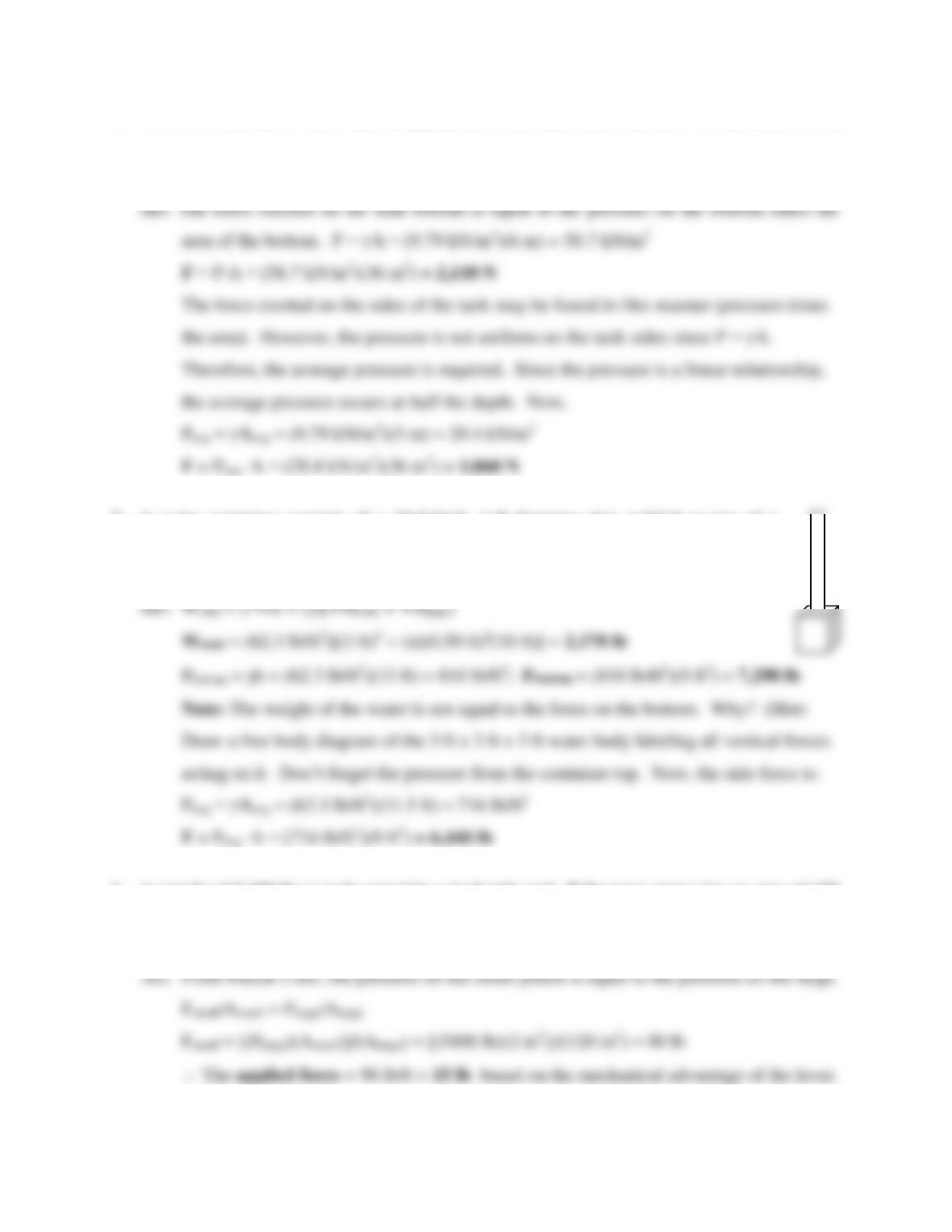4. A storage tank (6 m x 6m x 6m) is filled with water. Determine the force on the bottom and
on each side.
5. A water container consists of a 10-ft-high, 1-ft-diameter pipe welded on top of a
cube (3ft x 3ft x 3ft). The container is filled with water (20°C). Determine the weight
of the water and the pressure forces on the bottom and sides of the bottom cube.
6. A weight of 5,400 lbs is to be raised by a hydraulic jack. If the large piston has an area of 120
in.2 and the small piston has an area of 2 in.2, what force must be applied through a lever
having a mechanical advantage of 6 to 1?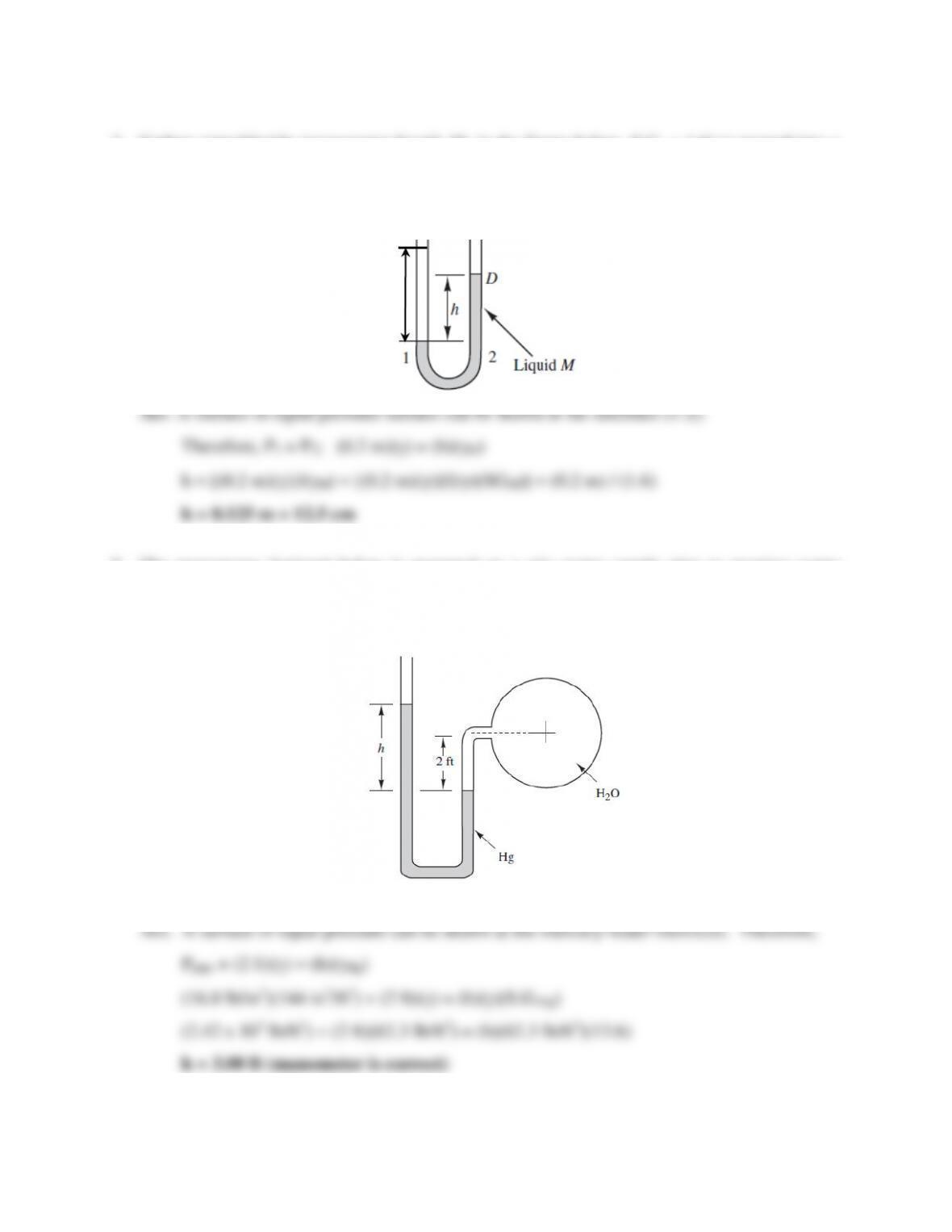7
7. Carbon tetrachloride (manometer liquid, M, in the figure below, S.G. = 1.6) is poured into a
U-tube with both ends open to the atmosphere. Then water is poured into one leg of the U-
tube until the water column (y) is 20 cm high. Determine the height of the carbon
tetrachloride (h) above the water-carbon tetrachloride interface (point 1).
8. The manometer depicted below is mounted on a city water supply pipe to monitor water
pressure. However, the field engineer suspects the manometer reading of h = 3 feet (Hg) may
be incorrect. If the pressure in the pipe is measured independently and found to be 16.8
lb/in.2 (psi), determine the correct value of the reading h.
y
Note: y = height of
water. Air is above
the Hg at point D.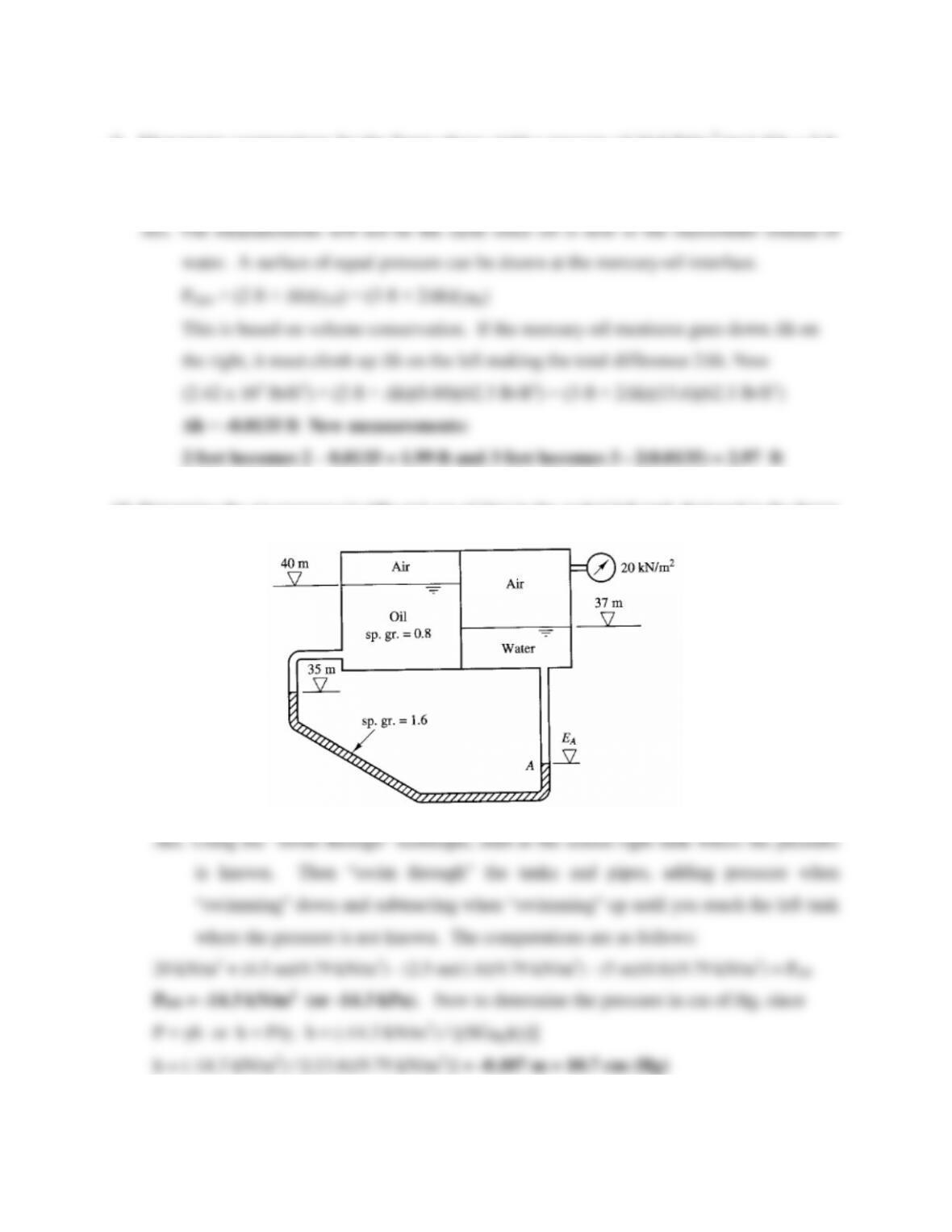8
9. Manometer computations for the figure above yield a pressure of 16.8 lb/in.2 (psi) if h = 3 ft.
If the fluid in the pipe was oil (S.G. = 0.80) under the same pressure, would the manometer
measurements (2 ft and 3 ft) still be the same? If not, what would the new measurements be?
10. Determine the air pressure (in kPa and cm of Hg) in the sealed left tank depicted in the figure
below if EA = 32.5 m.

## Trusted by Thousands ofStudents

Here are what students say about us.